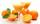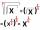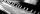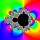# Sandpile

Auto sprinkled with sand to an approximately conical shape. Workers wanted to determine the volume (amount of sand) and therefore measure the circumference of the base and the length of both sides of the cone (over the top).

What is the volume of the sand cone, if the circumference of the base is 6 m and length of the two sides together 6 m?

Result

volume is:  2.7 m3

#### Solution:Leave us a comment of example and its solution (i.e. if it is still somewhat unclear...):Be the first to comment!#### To solve this example are needed these knowledge from mathematics:

Tip: Our volume units converter will help you with converion of volume units.

## Next similar examples:

1. Bottles of juiceHow many 2-liter bottles of juice need to buy if you want to transfer juice to 50 pitchers rotary cone shape with a diameter of 24 cm and base side length of 1.5 dm.
2. GasholderThe gasholder has spherical shape with a diameter 20 m. How many m3 can hold in?
3. Cross-sections of a coneCone with base radius 16 cm and height 11 cm divide by parallel planes to base into three bodies. The planes divide the height of the cone into three equal parts. Determine the volume ratio of the maximum and minimum of the resulting body.
4. Truncated coneCalculate the height of the rotating truncated cone with volume V = 1115 cm3 and a base radii r1 = 7.9 cm and r2 = 9.7 cm.
5. RotationRight triangle with legs 14 cm and 20 cm rotate around longer leg. Calculate the volume and surface area of the formed cone.
6. CalculationHow much is sum of square root of six and the square root of 225?
7. Theorem proveWe want to prove the sentence: If the natural number n is divisible by six, then n is divisible by three. From what assumption we started?
8. Square rootsWhat is equal to the product of the square roots of 295936?
9. BottlesThe must is sold in 5-liter and 2-liter bottles. Mr Kucera bought a total of 216 liters in 60 bottles. How many liters did Mr. Kucera buy in five-liter bottles?
10. Cube cornersFrom cube of edge 14 cm cut off all vertices so that each cutting plane intersects the edges 1 cm from the nearest vertice. How many edges will have this body?
11. PowerNumber ?. Find the value of x.
12. PianoIf Suzan practicing 10 minutes at Monday; every other day she wants to practice 2 times as much as the previous day, how many hours and minutes will have to practice on Friday?
13. TetrahedronCalculate height and volume of a regular tetrahedron whose edge has a length 18 cm.
14. ProductThe product of two consecutive odd numbers is 8463. What are this numbers?
15. Geometric progressionFill 4 numbers between 4 and -12500 to form geometric progression.Solve quadratic equation: 2x2-58x+396=0Three walls of the same cuboid has content 6 cm2, 10 cm2 and 15 cm2. Calculate the volume of the cuboid.